## 9.1 A Favorite Rule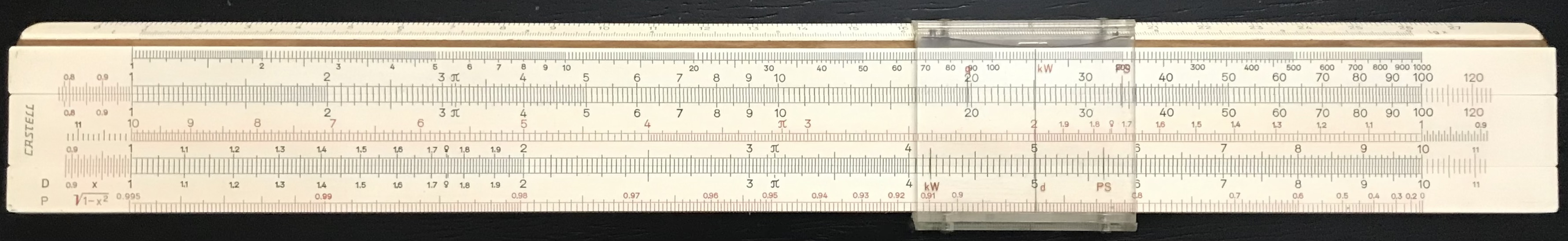The Faber-Castell Model 1/54 shown above has become one of my favorite all-purpose slide rules. The one I have in the collection was made in 1960, with tables on the back written in German, and has a very nice, solid “feel” to it. This one rule, with its standard 25 cm (10 inch) C/D scales, actually has a total of 13 computational scales, a single 7-hairline cursor, as well as a 27 cm measuring scale on the bottom edge of one side.

Except for the two scales at the bottom, the other scales on the front of the rule are not labeled. However, the standard scales are easy to identify: the K scale is at the top, followed by the usual A and B scales, a CI scale in the middle, followed by the C and D scales; then there is a P scale at the bottom of the rule, which gives $$\sqrt{1-x^2}$$, where $$x$$ is read on the D scale. See Slide Rule ABC’s and D’s for more information on all the scales.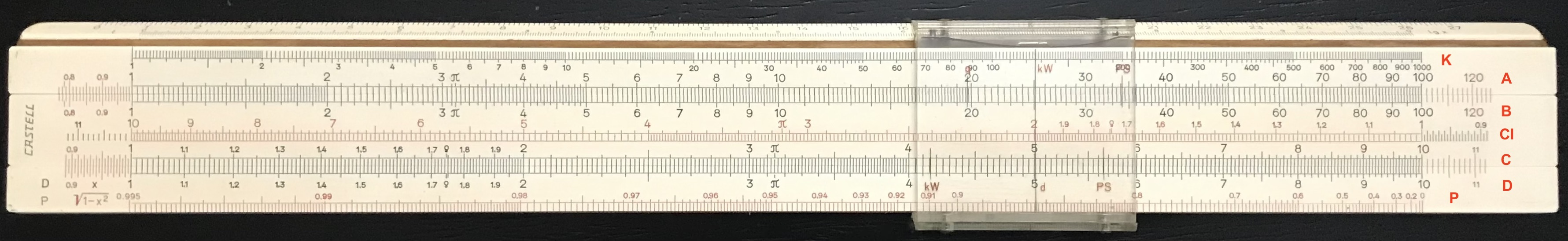A total of 5 hairlines appear on the front of the cursor. Besides the main hairline for primary use, the other four can be used for quick common calculations. For instance, if the main hairline (labeled d) is indicating the diameter of a circle, then the upper left short hairline (labeled q) gives the area of the circle. The other hairlines, labeled PS and kW give conversions between horsepower and kilowatts, respectively, in power calculations. (1 HP = 0.746 kW.) Note that a “conversion” is the multiplication by a factor, which is simply a particular offset on a log scale.

The cursor extends beyond the face of the rule to cover the top and bottom sides as well, and includes separate hairlines for reading scales located on the sides bringing the total number of hairlines to 7 on this rule. For trigonometry, a sine/cosine scale from $$5.8^\circ$$ to $$90^\circ$$ is on the side at the bottom of the rule. When the hairline is aligned with one of the black numbers, that angle’s sine is read on the D scale; if the red numbers are used, the D scale displays that angle’s cosine. Just below the sine/cosine scale is a tangent/cotangent scale that works similarly for angles between $$5.8^\circ$$ and $$45^\circ$$.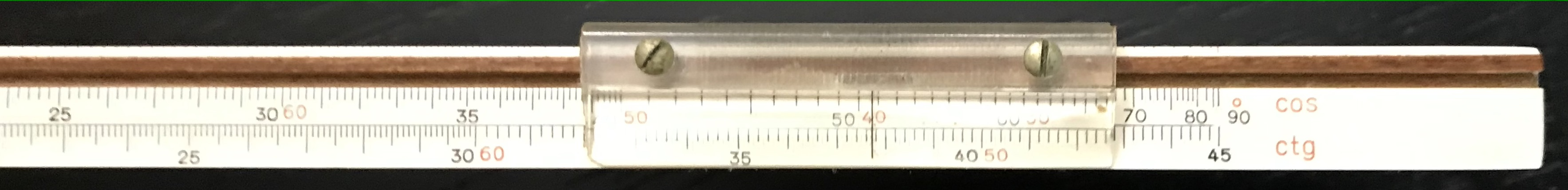On the upper side of the rule, along its edge, the centimeter scale can be used for measurements; next to this is an L scale (labeled $${\rm lg~x}$$ here) for finding common logarithms of the numbers along the D scale.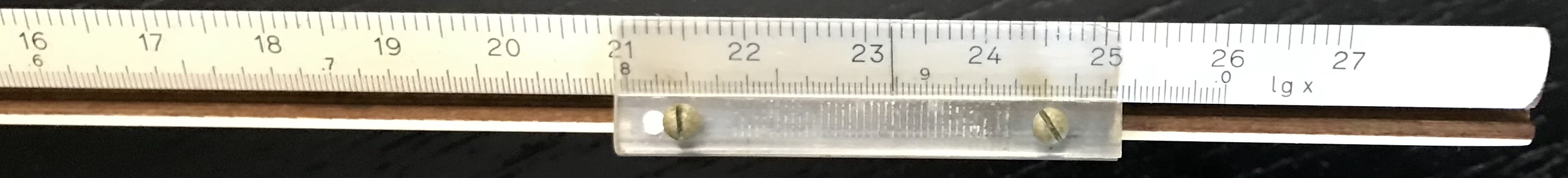If a rule contains a P scale on the front along with the usual scales (A/B, C/D, K, etc.), and three log-log scales on the back of the slide, as this one does, then it is called a Darmstadt scale set. Turning this rule over, we see a table of formulas, conversion factors and constants, but in addition we see two windows that allow us to read another set of scales – the values of the exponential function, $$e^x$$, where $$x$$ is read on the D scale and $$e$$ = 2.71828…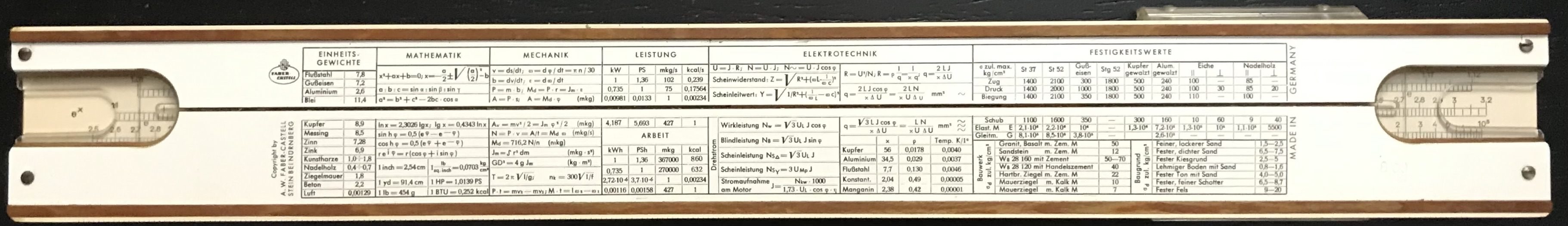For this rule, if the number on the D scale corresponds to a number in the range $$1<x<10$$, then the bottom exponential scale will give $$e^x$$; if the number on D lies in the range $$0.1<x<1$$, then the middle exponential scale is used; and if $$0.01<x<0.1$$, then the top exponential scale is used. With this scale (or set of 3 scales; the total length is 75 cm) one can find numbers raised to powers, as well as values of exponentials and, when used in reverse, the natural logarithms of numbers, and can also be used to compute hyperbolic trigonometric functions and so on.Close-up of the $$e^x$$ scale.

With the complete set of scales on this slide rule almost any every-day calculation for a scientist or engineer can be performed, making the Model 1/54 a beautiful and versatile computing device.